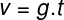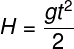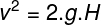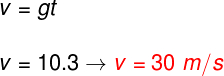Mechanics

# Free fall

Free fall is a movement in which bodies that are dropped at a certain height are accelerated by gravity towards the ground. In free fall, the effect of air resistance is disregarded, so in this type of movement, the falling time of objects does not depend on their mass or size, but only on the height at which they were released and the magnitude of the drop. Gravity acceleration in place. Free fall is uniformly accelerated , one-dimensional motion whose acceleration is the acceleration of gravity .

## free fall experiment

The most famous free fall experiment is the one that is often attributed to Galileo Galilei , although it is nothing but a legend, this experiment was very important for us to understand that the weight of bodies does not affect their fall time, in the event that the resistance offered by the air can be neglected.

According to the story, Galileo dropped objects of different masses from the top of the Leaning Tower of Pisa and concluded that the times of fall were equal . However, the experiment that was actually conducted by the Italian physicist involved an inclined plane on which different bodies were made to slide across its surface.

## Free fall formulas

The formulas used for free fall take into account, in most cases, a frame of reference that is in the same initial position as the falling object . We consider free fall as the movement when an object is released or released from rest (initial velocity equal to zero) from a certain height above the ground, in a region where there is gravitational acceleration. The cases in which objects start their movement with initial speeds different from zero, we say that they are vertical launches.

The formula that determines the speed of fall of a body that falls from rest is quite simple, check it out:v – Falling speed (m/s)

g – gravity (m/s²)

t – fall time (s)

The formula above indicates that the velocity acquired by the body can be calculated through the product of gravity and its time of fall.

To relate height and time, we use the following formula:H – height (m)

Analyzing the above equation, it is possible to see that the vertical distance traveled by a body in free fall is proportional to the square of the time. This indicates that at each instant the body will be falling a greater space, because its motion is accelerated.

There is also an equation that is able to relate the speed of fall to the height. This equation derives from Torricelli’s equation :## Examples of free fall

Check out some situations in which we can consider that the movement can be approximated to a free fall:

• Apple falling from a tree
• Cell phone falling to the floor
• A book falling off a shelf
• A glass falling from the table

In general, we can say that objects that fall from very small distances in relation to the ground describe a movement very close to that which would occur without the presence of air.

## Solved free fall exercises

For all the exercises below, consider g = 10 m/s²

Question 1) A 100 kg anvil is dropped from the top of a 20 m high building and falls towards the ground. Determine the speed at which the anvil is just before it touches the ground. Disregard air resistance.

a) 1 s

b) 2 s

c) 4 s

d) 3 s

e) 6 s

Template : Letter B

Resolution:

We will use the formula that relates height to fall time to solve this exercise, note:

Applying the data provided by the exercise to the formula, we find that the time to fall to a height of 20 m on Earth, where gravity is 10 m/s², is 2 s. The exercise mass makes no difference in the calculation, as air resistance is disregarded in this case.

Question 2) Two bodies of different masses, m 1 in 2 , where m 1 > m 2 , are abandoned at a certain height in a controlled place, from which all the air present has been removed. In relation to the movement described by the bodies, mark the correct alternatives.

I – The object of mass m 1 reaches the ground before the object of mass m 2.

II – The weight of the two bodies is equal.

III – The acceleration suffered by the two bodies is equal.

IV – The speed with which the body of mass m 1 reaches the ground is greater.

Are correct:

a) Only II.

b) I, II and III.

c) Only III.

d) I and II.

e) III and IV.

Template : Letter C

Resolution:

Let’s analyze the statements:

I – FALSE – As stated in the exercise statement, all the air was removed from the environment, so the fall time of the two bodies depends only on the height at which they were released and the local gravity.

II – FALSE – Weight is the measure of the force that the Earth exerts on bodies, due to its gravity. This force can be calculated as the product of mass and gravity, so the weights of the bodies are different. However, their masses are also different, this makes the acceleration acquired by them during the fall to be the same.

III – TRUE – Although the weight of bodies is different, their masses are also different. The ratio between these quantities has a constant magnitude, so gravity is the same for both.

IV – FALSE – As explained in the previous alternatives, the accelerations of the two bodies are the same, therefore, they will reach the ground at the same time.

Therefore, the only correct alternative is the letter C.

Question 3) Determine the speed with which an object hits the ground if it is in a free-fall motion that lasts a total of 3.0 s. Express your answer in km/h.

a) 40 km/h

b) 72 km/h

c) 36 km/h

d) 108 km/h

e) 30 km/h

Template : Letter D

Resolution:

Let’s calculate the velocity by multiplying gravity by the time of fall:After performing the above calculation, it is necessary to convert the speed to the unit km/h, for this, we simply multiply the result by the factor 3.6, resulting in a speed of 108 km/h.

Question 4) A 2 kg hammer is dropped on the Earth’s surface and is later dropped on the Moon at the same height. Regarding the falling movements in the two situations described, mark the correct alternative.

a) The weight of the hammer is equal on the Moon and Earth.

b) On the Moon, the hammer would be stationary in the air, because there is no gravity there.

c) The fall time is the same in both cases.

d) The weight of the hammer is greater on the Moon.

e) The time for the hammer to fall is longer on the Moon, because gravity is less there than on Earth.

Template : Letter E

Resolution:

The Moon’s gravity is less than Earth’s gravity, so the hammer’s weight is greater on Earth, in addition, the fall time on the Moon’s surface is longer.